# How to Apply Ohm's Law?

Zoey

## Homework Statement

Apply Ohm's law and the nature of parallel circuits to determine the total current in a parallel circuit with three resistors: 3Ω, 6Ω, and 9Ω, respectively. The total voltage in the circuit is 12V. Explain your reasoning.

[/B]
Ohm's Law

## The Attempt at a Solution

[/B]
I tried to plug the values into Ohm's law, but I don't think that is how I am supposed to go about this.

I am not asking for someone to give me the answer outright, just a place to start here!
All answers will be appreciated!Homework Helper
Gold Member
I tried to plug the values into Ohm's law, but I don't think that is how I am supposed to go about this.
It is necessary to show your working. Did you calculate their equivalent resistance first or did you directly apply Ohm's law? Both ways work fine.

Zoey
It is necessary to show your working. Did you calculate their equivent resistance first or did you directly apply Ohm's law? Both ways work fine.

Hi,
I just tried to apply Ohm's Law directly. I realized it wasn't going to work, because I have more values than are in that formula. But here is as far as I got.
First I added up all of the Ohms and got 18Ω. Then, I plugged it into the formula,

V/R = 12/18 = 0.6666666667

And I guess that could be right, I just figured that because of how I went about adding up the Ohms that it wasn't.

Thanks!

Staff Emeritus
Homework Helper
Hi,
I just tried to apply Ohm's Law directly. I realized it wasn't going to work, because I have more values than are in that formula. But here is as far as I got.
First I added up all of the Ohms and got 18Ω. Then, I plugged it into the formula,

V/R = 12/18 = 0.6666666667
Why would you add the resistance values together when you are told specifically that the resistors are in parallel?

Do you not understand what "parallel" means in this context?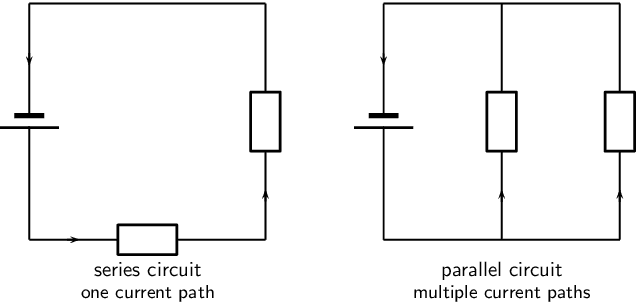Zoey
Why would you add the resistance values together when you are told specifically that the resistors are in parallel?

Do you not understand what "parallel" means in this context?As I said, I did not think I was doing it correctly. It was simply me attempting to go about the problem, not really having any idea how. I guess what I am really asking is how I would use this information in a formula.

Homework Helper
Gold Member
As I said, I did not think I was doing it correctly. It was simply me attempting to go about the problem, not really having any idea how. I guess what I am really asking is how I would use this information in a formula.
Have you studied the concept of equivalent resistance of a series/parallel network?

Zoey
Have you studied the concept of equivalent resistance of a series/parallel network?
No. That is what I was trying to get a grasp of.

Homework Helper
Gold Member
No. That is what I was trying to get a grasp of.
Ok. What do you understand from the fact that they are in parallel?

Zoey
Ok. What do you understand from the fact that they are in parallel?

I understand that by parallel it means there are multiple resistors that are side by side next to each other. What I am not understanding is how to apply this information to use it in a formula..

Homework Helper
Gold Member
I understand that by parallel it means there are multiple resistors that are side by side next to each other.
What can you say about voltage across parallel components?

Zoey
What can you say about voltage across parallel components?

I think I have figured it out, using the formula for total resistance.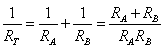= 3x6x9/3+6+9 = 162/8 = 9
Then I used the formula for total current: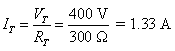12/9= 1.33333333333333

Homework Helper
Gold Member
I think I have figured it out, using the formula for total resistance.= 3x6x9/3+6+9 = 162/8 = 9
Then I used the formula for total current:12/9= 1.33333333333333
No. The equivalent resistance of parallel combination of 3Ω, 6Ω and 9Ω is not 9Ω.
The formula in your image is true for only two resistors in parallel.

Zoey
No. The equivalent resistance of parallel combination of 3Ω, 6Ω and 9Ω is not 9Ω.
The formula in your image is true for only two resistors in parallel.
Oops I think I used the wrong formula. Here is the formula for multiple resistors: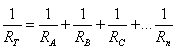Which would make 18, correct?

So then 12/18 is 0.666666667.

Homework Helper
Gold Member
Have you studied the theory behind series and parallel components in a circuit? Do you understand what happens to voltage or current when components are in series or parallel?
Oops I think I used the wrong formula. Here is the formula for multiple resistors:Which would make 18, correct?

So then 12/18 is 0.666666667.
No. Equivalent resistance of resistances in parallel is less than the least of them.

Zoey
Thank you for trying to assist me with this, and sorry if I don't seem to be understanding this. Could you tell me what formula is supposed to be used here then if it wasn't the one I used above?

Homework Helper
Gold Member
Thank you for trying to assist me with this, and sorry if I don't seem to be understanding this. Could you tell me what formula is supposed to be used here then if it wasn't the one I used above?
Well, the formula in your above post is correct but it doesn't give 18 ohm. Try again using that formula.

Zoey
What does 1/Rn stand for/mean though? Because all of the values I have are the resistors and the voltage...

Homework Helper
Gold Member
What does 1/Rn stand for/mean though? Because all of the values I have are the resistors and the voltage...
1/Requivalent...

Zoey
1/Requivalent...
Does that mean you would add all of the resistors + the equivalent of all of the resistors? So then it would be 36?

Skyrocket
I will give you a couple of hints.

One. For resistors in parallel the resistance of the combined resistors is less than the smallest resistor.

Two. The smallest resistor is 3 ohms. The current through that resistor at 12 volts is 4 amps.

Therefor the parallel resistance of all the resistors is less than 3 ohms, and the combined current will be greater than 4 amps.

•Zoey
Staff Emeritus
Homework Helper
Does that mean you would add all of the resistors + the equivalent of all of the resistors? So then it would be 36?
$$\frac{1}{a}+\frac{1}{b}+\frac{1}{c}≠\frac{1}{a+b+c}$$

You should review how to add fractions together.

Homework Helper

## Homework Statement

Apply Ohm's law and the nature of parallel circuits to determine the total current in a parallel circuit with three resistors: 3Ω, 6Ω, and 9Ω, respectively. The total voltage in the circuit is 12V. Explain your reasoning.

You do not need the formula for the equivalent resistance. All resistors are connected directly to the terminals of the battery. What is the voltage across each resistor? Apply Ohm's law to calculate the current flowing through resistors R1, R2, R3, then add them up.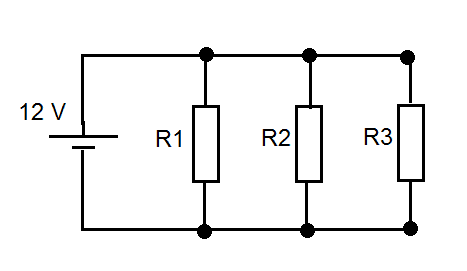•CWatters
late347
If you can calculate total resistance in parallel circuit. Then you are getting closer to the solution.

The other step is to know what is the total voltage in circuit with the parallel resistors.

Then you can find the total currwnt in circuit using ohms law.

The question only asked what is the total current. It is not needed to calculate each current at each resistor. (Because it was not asked to do so)

However that could still be done if you wanted to calculate that way... it would simply show that the current varies in those individual resistors compared to other resistors (in this scenario all resistors were 3 ohms 6 ohms 9 ohms.)

U=RI

I dont have expert qualifications to give advice but I was doing these problems yesterday myself hehe...

•Zoey
David Lewis
The question only asked what is the total current. It is not needed to calculate each current at each resistor. (Because it was not asked to do so)

Very true. The problem doesn't ask for the current through each resistor, but it's easy to calculate and, when you simply add the three currents together, you have your answer.

late347
Very true. The problem doesn't ask for the current through each resistor, but it's easy to calculate and, when you simply add the three currents together, you have your answer.

if you know for sure....

Do you actually get the total current, by using the ohms law, When we already know the total resistance and the total voltage in parallel circuit

In other words does the total current and total resistance yield us the total current ?

David Lewis
Yes. Absolutely. But if you want to find the answer quickly, you may make intermediate calculations that yield the solution indirectly.

Staff Emeritus
Homework Helper
if you know for sure....

Do you actually get the total current, by using the ohms law, When we already know the total resistance and the total voltage in parallel circuit

In other words does the total current and total resistance yield us the total current ?

That's something of a tautology. I think you meant to ask if the total resistance and the voltage drop yield the total current.

Gold Member
Could you tell me what formula is supposed to be used here then if it wasn't the one I used above?

Instead of hunting for the right equation, try understanding the situation. This is what you'll be tested on.

In a parallel circuit, each item has the same voltage across it. Use that, and ##V=IR## to find the answers.

•